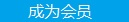您可以捐助，支持我们的公益事业。 1元 10元 50元 认证码：必填求知 文章 文库 Lib 视频 Code iProcess 课程 角色 咨询 工具 火云堂 讲座吧 建模者要资料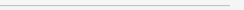订阅捐助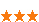424 次浏览     评价： 好 中 差
2018-11-15

 编辑推荐: 本文来自于segmentfault，文章详细介绍了如何在Python中构建机器学习分类器等相关知识。

Python 3 本地编程环境

 \$ . my_env/bin/activate

 (my_env) \$ python -c "import sklearn"

 Traceback (most recent call last): File "", line 1, in ImportError: No module named 'sklearn'

 (my_env) \$ pip install scikit-learn[alldeps]

 (my_env) \$ jupyter notebook

 ML Tutorial

 import sklearn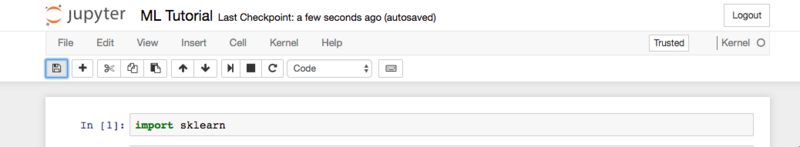Notebook

Scikit-learn安装了各种数据集，我们可以将其加载到Python中，并包含我们想要的数据集。导入并加载数据集：

 ML Tutorial

 ML Tutorial

 ... # Organize our data label_names = data['target_names'] labels = data['target'] feature_names = data['feature_names'] features = data['data']

 ML Tutorial

 ... # Look at our data print(label_names) print(labels) print(feature_names) print(features)ML Tutorial

 ... from sklearn.model_selection import train_test_split # Split our data train, test, train_labels, test_labels = train_test_split(features, labels, test_size=0.33, random_state=42)

 ML Tutorial

 ... from sklearn.naive_bayes import GaussianNB # Initialize our classifier gnb = GaussianNB() # Train our classifier model = gnb.fit(train, train_labels)

 ML Tutorial!

 ... # Make predictions preds = gnb.predict(test) print(preds)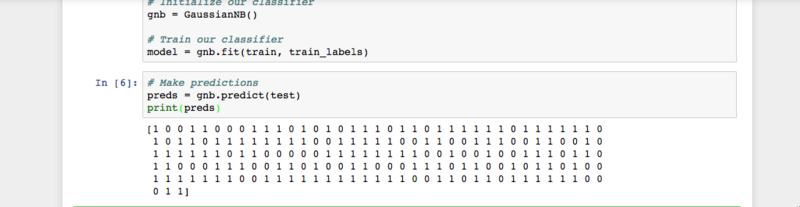ML Tutorial

 ... from sklearn.metrics import accuracy_score # Evaluate accuracy print(accuracy_score(test_labels, preds))ML教程

 from sklearn.datasets import load_breast_cancer from sklearn.model_selection import train_test_split from sklearn.naive_bayes import GaussianNB from sklearn.metrics import accuracy_score # Load dataset data = load_breast_cancer() # Organize our data label_names = data['target_names'] labels = data['target'] feature_names = data['feature_names'] features = data['data'] # Look at our data print(label_names) print('Class label = ', labels) print(feature_names) print(features) # Split our data train, test, train_labels, test_labels = train_test_split(features, labels, test_size=0.33, random_state=42) # Initialize our classifier gnb = GaussianNB() # Train our classifier model = gnb.fit(train, train_labels) # Make predictions preds = gnb.predict(test) print(preds) # Evaluate accuracy print(accuracy_score(test_labels, preds))424 次浏览  评价: 好 中 差订阅捐助
 相关文章 我们该如何设计数据库 数据库设计经验谈 数据库设计过程 数据库编程总结
 相关文档 数据库性能调优技巧 数据库性能调整 数据库性能优化讲座 数据库系统性能调优系列每天2个文档/视频 扫描微信二维码订阅
 订阅技术月刊 获得每月300个技术资源